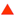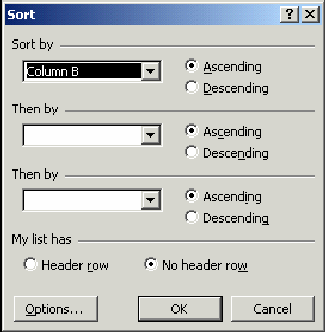# Computer application II (use of packages) windows, msword, powerpoint, excel and internet table of content chapter One

 Format à Column à Width command to display the Column Width dialog box Specify the width in the Column Width text box Click the OK button to close the Column Width dialog boxUsing Sorting Feature Excel enables you to sort data in rows or columns. You can sort data based on ascending or descending order. To sort data perform the following steps: Select the range of cells containing the data that need to be sorted Select the Data à Sort command to display the Sort dialog box Select the required options and click the OK button The Sort Dialog box options: Sort by: sort data by the specified column based on ascending or descending order. Then by: sort data by more than one column. Excel first sorts the column specified in the Sort By box and then by the columns specified in Then By boxes in sequence. My list has: This contains two radio buttons, Header row and No header row. If the first row has column labels, click the Header row radio button to exclude the first row from the sort. In contrast if you need to include the first row in the sort, you can click the No Header row radio button.Using formulas and functions At times there may be a need to perform some calculations on some numerical data that is represented in an Excel worksheet. To perform calculations, a formula can be used Formulas in Excel begin with an equal sign (=) E.g., =A1+A2+A3 is a formula to add the contents of cells A1, A2, and A3 The Formula bar is a bar at the top of the Excel window that displays the formula of the active cellThe Formula bar is displayed by default. You can choose not to view the Formula Bar. To stop viewing the Formula Bar, select View à Formula Bar command. You can later view the Formula Bar by selecting the same option. Entering Formulas in a Worksheet A formula can be typed in a cell to calculate and display the result of the formula in that cell. Eg., in a cell A1 a formula given as =B4+C5 adds the cell contents of cell B4 and cell C5 and display the result in cell A1 The formula specified must be preceded by an equal to (=) sign otherwise the calculation will not be performed Listed below are some examples of formulas: =35 * 44 =23 ^ 2 =C5 -C3 =C1+C2+C3+C4+C5 =S3 - D6 * 44 / 3 An important and useful feature of Excel is that it automatically recalculates the value of the cell that contains a formula if the values of the cells to which the formula refers to change. For example, the formula =C3+C4 in cell C5 is recalculated if the values of cells C3 or C4 change.Functions Excel provides many functions - mathematical, statistical, logical, etc. Mathematical functions Sum(), Product() E.g, to calculate the sum of values stored in cells C1 to C5, use =SUM(C1:C5). Statistical functions Average(), Max(), Min(), Count(), Round() Eg., to find the average of the values in cells C1 to C5, use =AVERAGE(C1:C5). Date and time functions Now(), Today(), Date(), Time() Eg., =TIME(9,0,0) returns 9:00 A.M and =TIME(20,15,30) returns 8:15 P.M. Logical functions If(), Not() E.g., For the formula =If(B2>70, “A”, “B”), a grade A is displayed if marks are above 70 and a grade B is displayed if marks are below or equal to 70.Introduction to Internet Introduction to Internet Objectives Internet and WWW Applications on the Internet Web terminology Navigating the Web sites Connecting a computer to the Internet Working with the Internet Explorer Using Help Printing Web pages Searching for information on the Internet Implementing securityInternet and WWW The Internet is a worldwide system of computer networks. Users can obtain information about any subject or topic by connecting to the Internet The Internet was first known as ARPANET and was started by the U.S. government in 1969 - Advanced Research Projects Agency (ARPA) The original aim of ARPANET was to create a network that would allow researchers working on computers at one university to connect to computers at other universities The original aim of ARPANET was to create a network that would allow researchers working on computers at one university to connect to computers at other universities The World Wide Web (WWW) also called the Web is a subset of the Internet The Web is a series of interconnected servers that support specially formatted documents Today, the Internet has become an important part of our day-to-day life The Internet is used today in diverse field - business, entertainment, education, communication, medicine, defence, engineering, and sports. The WWW also called the Web is a subset of the Internet The Internet and the World Wide Web are often used synonymously but they are different. The Web is a series of interconnected servers that support specially formatted documents This means you can switch from one document to another just by clicking on marked text. A server is a program or a computer that responds to requests from other programs and delivers the requested documentsApplications on the Internet The Internet provides various types of services, which include: